# Optic equations

## Diopter or Dioptre

The diopter unit is simply the inverse of the focal length in meters.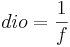Thus a +2 diopter lens has a focal length of 500mm

You can combine lenses of different diopters arranged in contact - thus a -2 diopter lens plus a +4 diopter lens would give us the same 500mm focal length.

## Focal Length

If a lens has surface radii of R1 and R2 and made of glass with an n refractive index the focal length, f, is defined below

R1 and R2 = surface radii (negative for convex lens surface)

ng = refractive index of the lens glass

f = focal length of lens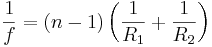## Depth of Field

Depth of Field is the minimum to maximum distance in focus.

## Contrast Ratio

Contrast ratio is the difference between the brightest and darkest point in an image.

## Lens power or Magnification ratio

Where

m = Magnification ratio or Power

hi = image height

ho = object height

Di = distance from lens to image

Do = distance from lens to object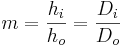## Spherometers - lens Radius from arc depth

C of the Triangle is the same as Radius.

The bottom has a calculation of the Spherometers leg from the radius.

## Wavelength of LED or Laser based on band-gap voltage

This is an estimation because the voltage drop is close to the band gap of a forward biased diode.

l = wave length in nm

Eg = band-gap voltage

then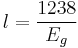Thus those red LEDs with a 1.9V drop run about 652 nm. (GaAs0.6P0.4)

This appears derived from ,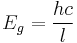Where:

h = Plank's Constant = 4.13 x 10-15 eV·s
c = speed of light = 2.998 x 108 m/s

## Colors of wave lengths

Infrared 710nm and goes down to roughly 2000nm.

The visible range is considered to be roughly 400nm to 710nm.

390nm to 420 is Blue (or Violet),

530nm is green

600nm to 710nm is considered red.

## Extreme objectives

If you found this information useful - all I ask is to look at our home page and see if we have any products that might be of use to you or a colleague. Link to us if you have a web page. If you have some thing to add post it here!

 Top Page wiki Index

## Disclaimer

This information may have errors; It is not permissible to be read by anyone who has ever met a lawyer.
Use is confined to Engineers with more than 370 course hours of electronic engineering for theoretical studies.
ph +1(785) 841-3089